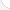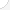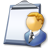Chemical engineers Description: Design chemical plant equipment and devise processes for manufacturing chemicals and products, such as gasoline, synthetic rubber, plastics, detergents, cement, paper, and pulp, by applying principles and technology of chemistry, physics, and engineering.Source: Bureau of Labor Statistics, U.S. Department of Labor, Occupational Outlook Handbook, 2008-09 Edition at http://www.bls.gov/OCO/Complete Job Profile: http://www.bls.gov/oco/ocos027.htmSalary: \$50,001 or more per yearComments:I am an Analytical chemist at a biopharmaceutical company. I use it to calculate concentrations for buffers and sample preparation. I use it to calculate the kinetic energy and heat imparted to drug components during formulation to determine the effects of heat inactivation based on different rotational speeds. I use to process data. But most importantly, I use it to when devising investment schemes to make a profit off the money I set aside for a future retirement to have a seven figure 401K and savings/stocks. Explain that to your students. Or they can be ignorant and calculate how much time they can take off their sentence by good behavior. Math is important to everyone. It is the very foundation of all true science!

There are 59 math topics Chemical engineers need to know.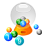Basic Math / Algebra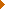FractionsDecimalsRatio and ProportionPercentCustomary MeasurementMetric MeasurementMeasurement ConversionBasic ProbabilityBasic StatisticsStatistical GraphingPowers and RootsOther Number BasesNegative NumbersScientific NotationBasic Problem Solving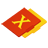First-Year AlgebraUsing FormulasLinear EquationsOperations with PolynomialsFactoring PolynomialsRational ExpressionsCoordinate Graphing 2DLinear SystemsQuadratic EquationsAlgebraic Representation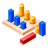GeometryBasic TerminologyAngle MeasurementSimilarityGeometric MeanPythagorean TheoremRight Triangle TrigonometryCirclesConstructionsAreaVolumeTransformationsMake/Use 3D Drawings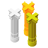Second-Year Algebra / TrigonometryFunctionsVariationPolynomial EquationsLogarithmsMatricesCoordinate Graphing 3DAdvanced StatisticsTrigonometric/Circular FunctionsGraphs of Trigonometric FunctionsTrigonometric IdentitiesTrigonometric Equations/InversesPolar Coordinates/GraphsVectors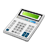Other TopicsCalculus and Higher MathBasic Calculator UseScientific Calculator UseComputer UseComputer ProgrammingGroup Problem SolvingMental MathInductive/Deductive ReasoningMath CommunicationsMathematical Modeling